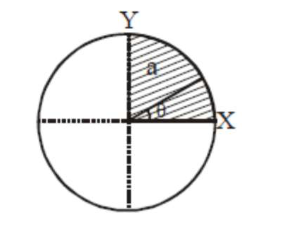# The disc of mass M with uniform surface mass densityQuestion:

The disc of mass $M$ with uniform surface mass density $\sigma$ is shown in the figure. The centre of mass of the quarter disc (the shaded area) is at the position $\frac{x}{3} \frac{a}{\pi}, \frac{x}{3} \frac{a}{\pi}$ where $x$ is_____________

(Round off to the Nearest Integer)

[a is an area as shown in the figure]Solution:

(4)

C.O.M of quarter disc is at $\frac{4 \mathrm{a}}{3 \pi}, \frac{4 \mathrm{a}}{3 \pi}$

$=4$